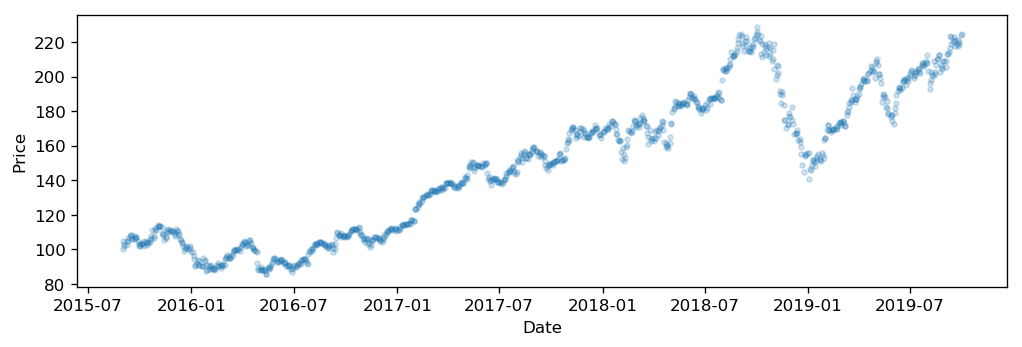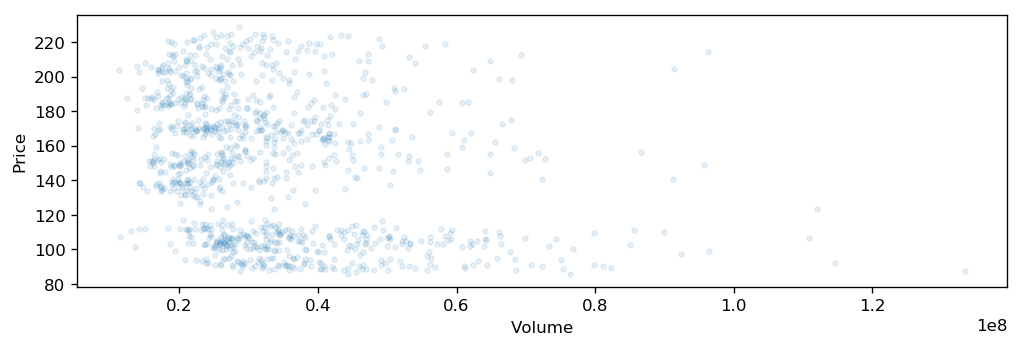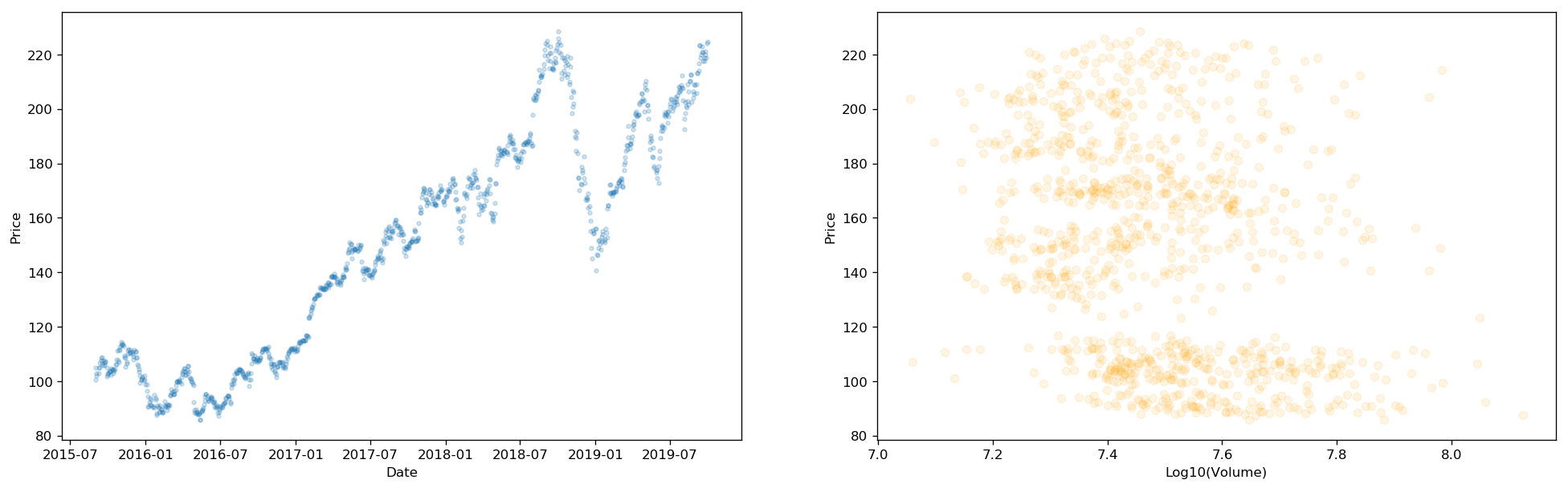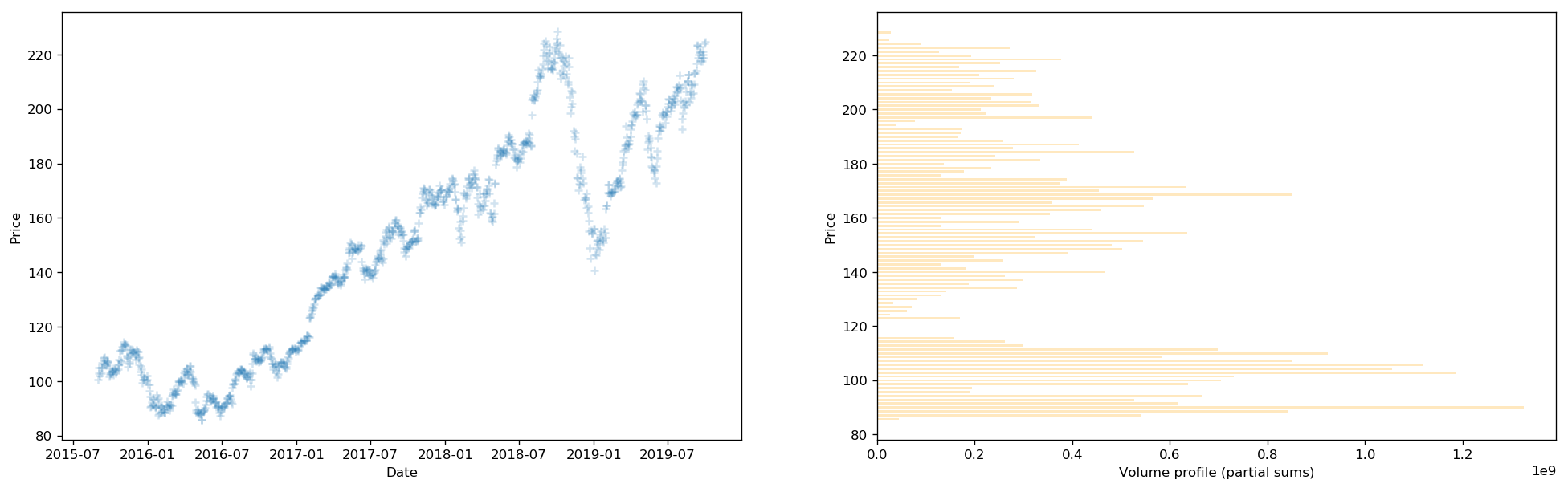## Volume Profile for stocks in python (VPVR indicator, Volume Profile Visible Range)¶

In this post we will see how to compute volume profile for given stock in python.

Motivation:

• This is paid feature on tradingview.com, it is called VPVR indicator (Volume Profile Visible Range). Yet computation in python is actually rather straightforward.
• Volume profile might help us detect support and resistance levels that can theoretically serve as good entry or exit points in trading. Confluence with other indicators such as RSI, stochastic (or any other basic indicator really) is preffered.

See below link for more in depth explanation of VPVR indicator: https://www.tradingview.com/wiki/Volume_Profile

We will get the stock data from Yahoo finance API.

In :
#optional installations:

from IPython.core.display import display, HTML
display(HTML("<style>.container { width:100% !important; }</style>"))

# ___library_import_statements___
import pandas as pd

# for pandas_datareader, otherwise it might have issues, sometimes there is some version mismatch
pd.core.common.is_list_like = pd.api.types.is_list_like
import numpy as np
import matplotlib.pyplot as plt
import seaborn as sns
import datetime
import time

import yfinance as yahoo_finance

#maybe I dont need this line
#yahoo_finance.pdr_override()

%matplotlib inline

In :
# ___variables___
ticker = 'AAPL'
start_time = datetime.datetime(2015, 9, 1)
#end_time = datetime.datetime(2019, 1, 20)
end_time = datetime.datetime.now().date().isoformat()         # today

In :
# yahoo gives only daily historical data
connected = False
while not connected:
try:
df = web.get_data_yahoo(ticker, start=start_time, end=end_time)
connected = True
print('connected to yahoo')
except Exception as e:
print("type error: " + str(e))
time.sleep( 5 )
pass

# use numerical integer index instead of date
df = df.reset_index()

connected to yahoo
Date        High         Low        Open       Close      Volume  \
0 2015-09-01  111.879997  107.360001  110.150002  107.720001  76845900.0
1 2015-09-02  112.339996  109.129997  110.230003  112.339996  61888800.0
2 2015-09-03  112.779999  110.040001  112.489998  110.370003  53233900.0
3 2015-09-04  110.449997  108.510002  108.970001  109.269997  49996300.0
4 2015-09-08  112.559998  110.320000  111.750000  112.309998  54843600.0

0  100.533249
1  104.845024
2  103.006447
3  101.979836
4  104.817017

In :
df.rename(columns={'Adj Close': 'Adj_Close'}, inplace=True)

        Date        High         Low        Open       Close      Volume  \
0 2015-09-01  111.879997  107.360001  110.150002  107.720001  76845900.0
1 2015-09-02  112.339996  109.129997  110.230003  112.339996  61888800.0
2 2015-09-03  112.779999  110.040001  112.489998  110.370003  53233900.0
3 2015-09-04  110.449997  108.510002  108.970001  109.269997  49996300.0
4 2015-09-08  112.559998  110.320000  111.750000  112.309998  54843600.0

0  100.533249
1  104.845024
2  103.006447
3  101.979836
4  104.817017


Let's visualise price and corresponding transaction volume.

#### Price Chart¶

In :
plt.figure(figsize=(10, 3), dpi= 120, facecolor='w', edgecolor='k')
plt.xlabel('Date')
plt.ylabel('Price')

Out:
Text(0, 0.5, 'Price')#### Volume distribution¶

In :
plt.figure(figsize=(10, 3), dpi= 120, facecolor='w', edgecolor='k')
plt.xlabel('Volume')
plt.ylabel('Price')

Out:
Text(0, 0.5, 'Price')We can see some clusters a little bit. This points out that some price levels are being transacted more often than others. Price range that has the highest volume might not be very clear though from this graph.

Let's compute partial sums of volume for price range. Once we have aggregated volume for multiple price ranges, we can then plot a histogram of the partial volumes. This should give us clearer picture.

Below few lines are just some pandas acrobatics for quick checks and a bit of troubleshooting:

In :
sub_df = df.loc[df['Adj_Close'].between(24, 25, inclusive=False)]

In :
df.iloc['Adj_Close']

Out:
103.63176727294922
df.iloc['Volume']
In :
sub_df.index.values

Out:
array([], dtype=int64)

Computation of partial volumes:

In :
start_price = df['Adj_Close'].min()

low = start_price
# delta means granularity in volume aggregation range, it is delta in price
# the volume corresponds to price
delta = (stop_price - start_price)/100    # here we are splitting whole price range into blocks
high = 0

idx_array = []
vol_array = []
low_array = []

while high < stop_price:
volume = 0
high = low + delta

low_array.append(low)

for i in sub_df.index.values:
#print(i)
#print(df.iloc[i]['Volume'])
volume = volume + df.iloc[i]['Volume']
#print('total partial volume: ', volume)

vol_array.append(volume)
low = high

#print('final vol_array: ', vol_array)

for idx, var in enumerate(vol_array):
#print("{}: {}".format(idx, var))
idx_array.append(idx)


Price range in selected time frame:

In :
#minimum price
print('start_price', start_price)

#maximum price
print('stop_price', stop_price)

start_price 85.65148162841797
stop_price 228.52381896972656

In :
print(high)

229.95254234313927


### Final visualization:¶

We will plot 2 pairs of graphs.

• price and corresponding volume
• price and histogram of the partial volumes for given partial price range (price range is in code marked as "delta" variable)

Histogram seems to be easier to interpret.

##### Price and volume¶
In :
#price and corresponding volume
plt.figure(figsize=(20, 20), dpi= 120, facecolor='w', edgecolor='k')

plt.subplot(321)
plt.xlabel('Date')
plt.ylabel('Price')

plt.subplot(322)
plt.xlabel('Log10(Volume)')
plt.ylabel('Price')

plt.show()##### Price and volume histogram¶
In :
#price and corresponding partial volume profile
plt.figure(figsize=(20, 20), dpi= 120, facecolor='w', edgecolor='k')

plt.subplot(321)
plt.xlabel('Date')
plt.ylabel('Price')

plt.subplot(322)
plt.barh(low_array, vol_array, alpha = 0.25, color='orange')
plt.xlabel('Volume profile (partial sums)')
plt.ylabel('Price')

plt.show()#### Summary:¶

Volume profile can be used as an indicator for a price at which market participants tend to buy or sell significant amounts of given stock. This might indicate possible support and resistance price levels.

In general, partial volumes tend to be locally peaking (having local maxima) for price levels where price is consolidating for a period of time or for significant local minima and local maxima in price (points where we should go long or go short).

Especially interesting might be loking at the correlation between volume profile and for example weekly RSI indicator values (or maybe weekly Stochastic RSI).